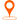您的位置：首页 > 技术观点 > 信息详情

# jquery实现的判断倒计时是否结束的代码

function done(){

var str=\$('#end').text();

var out=str.match(/d+/g);

console.log(out);

var h=parseInt(out),m=parseInt(out),s=parseInt(out);

console.log(h+'#'+m+'#'+s);

var calc=h*3600+m*60+s;

console.log(calc);

if(calc==0){

//code

else{

console.log('等待..');

var t=setTimeout('done()',1000);

done();

1.function done(){}，此函数实现判断倒计时结束效果。

2.var str=\$('#end').text()，获取指定元素中的文本内容，本代码中应该倒计时当前时间.

3.var out=str.match(/d+/g)，获取时间日期中数字的数组。

4.var h=parseInt(out),m=parseInt(out),s=parseInt(out)，分别获取小时、分钟和秒。

5.var calc=h*3600+m*60+s，转换成秒。

6.if(calc==0){//code}，判断倒计时是否结束，然后指定相应的操作。

7.var t=setTimeout('done()',1000)，每隔一秒执行一次判断函数。

8.done()，执行此函数。

jquery实现倒计时代码如下所示：

\$(function(){ var tYear = ""; //输入的年份 var tMonth = ""; //输入的月份 var tDate = ""; //输入的日期 var iRemain = ""; //开始和结束之间相差的毫秒数 var sDate = ""; //倒计的天数 var sHour = ""; //倒计时的小时 var sMin = ""; //倒计时的分钟 var sSec = ""; //倒计时的秒数 var sMsec = ""; //毫秒数 //通用工具函数，在个位数上加零，根据传的N的参数，来设前面加几个零 function setDig(num,n){ var str = ""+num; while(str.length<n){ str="0"+str } return str; } //获得相差的天，小时，分钟，秒 function getdate(){ //创建开始时间和结束时间的日期对象 var oStartDate = new Date(); var oEndDate = new Date(); //获取文本框的值 tYear = \$("#tyear").val(); tMonth = \$("#tmonth").val(); tDate = \$("#tdate").val(); //设置结束时间 oEndDate.setFullYear(parseInt(tYear)); oEndDate.setMonth(parseInt(tMonth)-1); oEndDate.setDate(parseInt(tDate)); oEndDate.setHours(0); oEndDate.setMinutes(0); oEndDate.setSeconds(0); //求出开始和结束时间的秒数(除以1000) iRemain = (oEndDate.getTime() - oStartDate.getTime())/1000; //总的秒数除以一天的秒数，再取出整数部分，就得出有多少天。 sDate = setDig(parseInt(iRemain/(60*60*24)),3); //总的秒数除以一天的秒数，然后取其中的余数，就是把整数天扣除之后，剩下的总秒数。 iRemain %= 60*60*24; //剩下的总秒数除以一个小时的秒数，再取整数部分，就是有多少小时。 sHour = setDig(parseInt(iRemain/(60*60)),2) //剩下的总秒数除以一个小时的秒数，再取其余数，这个余数，就是扣除小时这后，剩下的总秒数。 iRemain %= 60*60; //剩下的总秒数除以一分钟的秒数，再取其整数部分，就是有多少分钟。 sMin = setDig(parseInt(iRemain/60),2) //剩下的总秒数除以一分钟的秒数，再取其余数，这个余数，就是扣除分钟之后，剩下的总秒数。 iRemain%=60; //剩下的秒数 sSec = setDig(iRemain,2); //毫秒数 sMsec = sSec*100; } //更改显示的时间 function updateShow(){ \$(".showdate span").text(tYear+"-"+tMonth+"-"+tDate); \$(".count span").each(function(index, element) { if(index==0){ \$(this).text(sDate); }else if(index==1){ \$(this).text(sHour); }else if(index == 2){ \$(this).text(sMin); }else if(index == 3){ \$(this).text(sSec); }else if(index == 4){ \$(this).text(sMsec); } }); } //每一秒执行一次时间更新 function autoTime(){ getdate(); //如果小于零，清除调用自己，并且返回 if(iRemain<0){ clearTimeout(setT); return; } updateShow(); var setT = setTimeout(autoTime,1000); } //点击按钮开始计时 \$("button").click(function(){ autoTime(); }) })

1.取模运算：

iRemain %= 60*60*24;

2.工具函数 setDig(num,n)

• 1、

• 2、

• 3、

• 4、